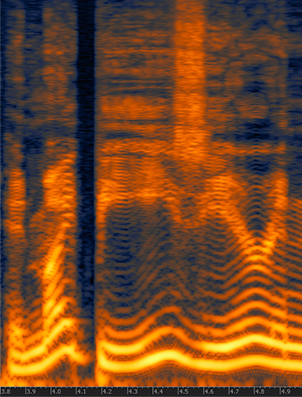# Listening to the neural network gradient norms during training

Training neural networks is often done by measuring many different metrics such as accuracy, loss, gradients, etc. This is most of the time done aggregating these metrics and plotting visualizations on TensorBoard.There are, however, other senses that we can use to monitor the training of neural networks, such as sound. Sound is one of the perspectives that is currently very poorly explored in the training of neural networks. Human hearing can be very good a distinguishing very small perturbations in characteristics such as rhythm and pitch, even when these perturbations are very short in time or subtle.

For this experiment, I made a very simple example showing a synthesized sound that was made using the gradient norm of each layer and for step of the training for a convolutional neural network training on MNIST using different settings such as different learning rates, optimizers, momentum, etc.

You’ll need to install PyAudio and PyTorch to run the code (in the end of this post).

### Training sound with SGD using LR 0.01

This segment represents a training session with gradients from 4 layers during the first 200 steps of the first epoch and using a batch size of 10. The higher the pitch, the higher the norm for a layer, there is a short silence to indicate different batches. Note the gradient increasing during time.

### Training sound with SGD using LR 0.1

Same as above, but with higher learning rate.

### Training sound with SGD using LR 1.0

Same as above, but with high learning rate that makes the network to diverge, pay attention to the high pitch when the norms explode and then divergence.

### Training sound with SGD using LR 1.0 and BS 256

Same setting but with a high learning rate of 1.0 and a batch size of 256. Note how the gradients explode and then there are NaNs causing the final sound.

### Training sound with Adam using LR 0.01

This is using Adam in the same setting as the SGD.

## Source code

For those who are interested, here is the entire source code I used to make the sound clips:

import pyaudio
import numpy as np
import wave

import torch
import torch.nn as nn
import torch.nn.functional as F
import torch.optim as optim
from torchvision import datasets, transforms

class Net(nn.Module):
def __init__(self):
super(Net, self).__init__()
self.conv1 = nn.Conv2d(1, 20, 5, 1)
self.conv2 = nn.Conv2d(20, 50, 5, 1)
self.fc1 = nn.Linear(4*4*50, 500)
self.fc2 = nn.Linear(500, 10)

self.ordered_layers = [self.conv1,
self.conv2,
self.fc1,
self.fc2]

def forward(self, x):
x = F.relu(self.conv1(x))
x = F.max_pool2d(x, 2, 2)
x = F.relu(self.conv2(x))
x = F.max_pool2d(x, 2, 2)
x = x.view(-1, 4*4*50)
x = F.relu(self.fc1(x))
x = self.fc2(x)
return F.log_softmax(x, dim=1)

def open_stream(fs):
p = pyaudio.PyAudio()
stream = p.open(format=pyaudio.paFloat32,
channels=1,
rate=fs,
output=True)
return p, stream

def generate_tone(fs, freq, duration):
npsin = np.sin(2 * np.pi * np.arange(fs*duration) * freq / fs)
samples = npsin.astype(np.float32)
return 0.1 * samples

def train(model, device, train_loader, optimizer, epoch):
model.train()

fs = 44100
duration = 0.01
f = 200.0
p, stream = open_stream(fs)

frames = []

for batch_idx, (data, target) in enumerate(train_loader):
data, target = data.to(device), target.to(device)
output = model(data)
loss = F.nll_loss(output, target)
loss.backward()

norms = []
for layer in model.ordered_layers:

tone = f + ((norm_grad.numpy()) * 100.0)
tone = tone.astype(np.float32)
samples = generate_tone(fs, tone, duration)

frames.append(samples)

silence = np.zeros(samples.shape * 2,
dtype=np.float32)
frames.append(silence)

optimizer.step()

# Just 200 steps per epoach
if batch_idx == 200:
break

wf = wave.open("sgd_lr_1_0_bs256.wav", 'wb')
wf.setnchannels(1)
wf.setsampwidth(p.get_sample_size(pyaudio.paFloat32))
wf.setframerate(fs)
wf.writeframes(b''.join(frames))
wf.close()

stream.stop_stream()
stream.close()
p.terminate()

def run_main():
device = torch.device("cpu")

transform=transforms.Compose([
transforms.ToTensor(),
transforms.Normalize((0.1307,), (0.3081,))
])),
batch_size=256, shuffle=True)

model = Net().to(device)
optimizer = optim.SGD(model.parameters(), lr=0.01, momentum=0.5)

for epoch in range(1, 2):

if __name__ == "__main__":
run_main()


## 10 thoughts to “Listening to the neural network gradient norms during training”

1.hady elsahar says:

cool stuff i wonder if debugging by listening could be a thing.

2.David says:

This is a great idea Christian! Our hearing is most sensible to textures and colors (wave shape and frequency balance), so maybe that’s an interesting direction to explore. I’ll definitely try this!

3.Vaibhav says:

Can this also be a way of how the gradients are flowing/updating through the dimensions?

1.Vaibhav says:

way of interpreting*

4.Firmino Changani says:

Great article Christian,

Even tough I am not a data scientist, you wrote the article in a way that even newbies could understand.

1.Christian S. Perone says:

Thanks for the feedback !!!

5.Roberto Chavez says:

You are a god

6.aba12 says:

This is really crazy..!!!

7.Marx Melencio says:

Hi. Really cool concept! Especially for engineers like myself. I’m a completely blind Deep Learning engineer. Since I started, I listen to training metrics as they’re read by my screenreader software while they’re printed in stdout. But this appears to be an optimum way to gain better intuition on what’s currently happening during the training process. And I can just use my screenreader at any point in time to read the numbers in stdout to verify and re-calibrate my abstractions. Now I just need to integrate this, though something with smoother “good-for-the-ears” audio, into Keras. Thanks for the idea!!!

This site uses Akismet to reduce spam. Learn how your comment data is processed.